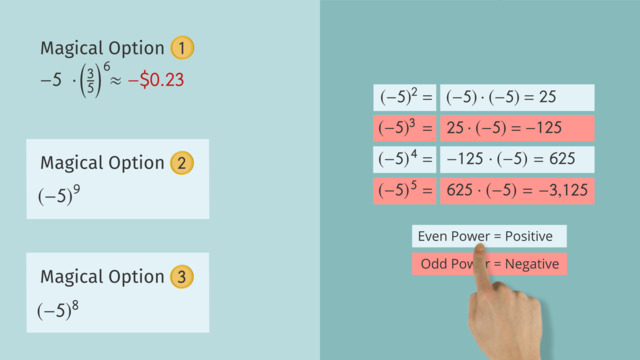# Exponential NotationRating

Be the first to give a rating!

The authorsChris S.

## Basics on the topicExponential Notation

After this lesson you will be able to use exponents, and determine whether a number raised to a given power will be positive or negative.

The lesson begins with the definition of exponential notation. This leads to an explanation of why negative numbers raised to an even power produce positive results, while negative numbers raised to an odd power produce negative results.

Learn about exponents by helping Wade and Skeeter decide what to do with the magical fish!

This video includes key concepts, notation, and vocabulary such as: base (the number being multiplied); exponent (the number of times the base is being multiplied by itself); the phrase, “x raised to the nth power” (where n is the exponent and x is the base); square (x raised to the second power is called the “square” of x); and cube (x raised to the third power is called the “cube” of x).

Before watching this video, you should already be familiar with exponential notation using base 10, and multiplication as repeated addition.

After watching this video, you will be prepared to learn about fractional or negative exponents.

Common Core Standard(s) in focus: 8.EE.A.1 A video intended for math students in the 8th grade Recommended for students who are 13-14 years old